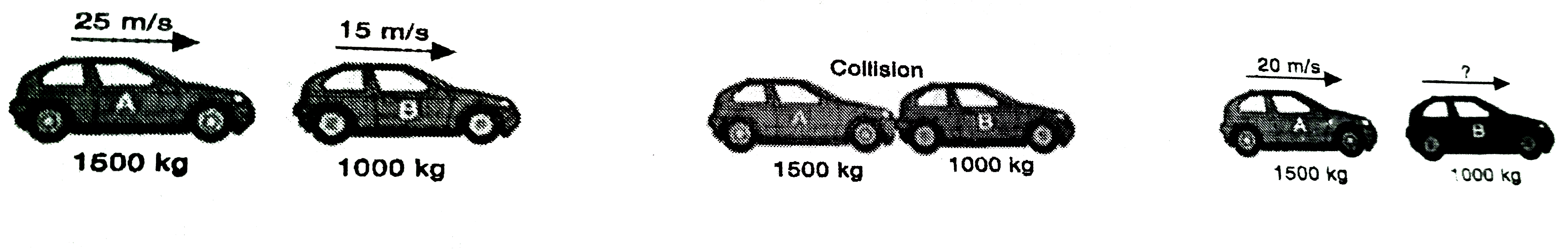Question

Updated on:30/05/2023

# The car A of mass 1500 kg, travelling at 25 m/s collides with another car B of mass 1000 kg travelling at 15 m/s in the same direction. After collision the velocity of car A becomes 20 m/s. Calculate the velocity of car B after the collision.Text Solution

Solution
In order to solve this problem, we will calculate the total momentum of both the cars, before and after the collision.
(a) Momentum of car A(before collision)=Mass ofcar A×Velocity ofcar A
=1500×25
=37500 kg.m/s
Momentum of car B(before collision)=Mass ofcar B×Velocity ofcar B
=1000×15
=15000 kg.ms
Total momentum of car A and car B=37500+15000
(before collision)=52500 kg.m/s
(b) After collision,the velocity of car A of mass 1500 kg becomes 20 m/s.
So, Momentum of car A=1500×20
(after collision)=30000 kg.m/s
After collision, suppose the velocity of car B of mass 1000 kg becomes v m/s
So, Momentum of car B=1000×v
(after collision)=1000 v kg.m/s
Total momentum of car A and B = 30000+1000 v.......(2)
(after collision)
Now, according to the law of conservation of momentum :
Total momentum=Total momentum
before collision after collision
That is, 52500=30000+1000 v
1000 v=52500-30000
1000 v=22500
v=225001000
v=22.5 m/s
Thus, the velocity of car B after the collision will be 22.5 m/s.